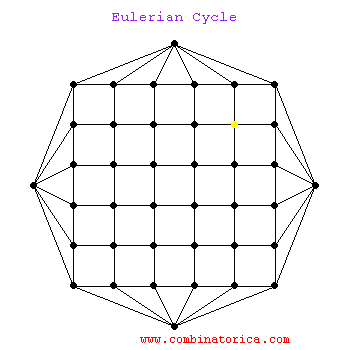# May 1st

Eulerian Circuits## Vocab

• a WALK on a graph is a sequence of vertex, edge, vertex, edge, ... , vertex where each edge joins the vertices surrounding it.
• LENGTH OF A WALK is the number of edges.
• a CLOSED WALK has first and last vertex as the same (OPEN WALK when they are different)
• a PATH is a walk in which every vertex is distinct
• TRAIL is a walk that never repeats an edge (can repeat vertices not edges)
• CIRCUIT is a closed trail (can repeat vertices but not edges)
• CYCLE is a circuit with only the first and last vertex equal (no repeated vertices or edges other than start and stop point).
• EULERIAN CIRCUIT is a circuit that contains every vertex and every edge.
• a graph is CONNECTED when there is a walk between any two vertices
• EULERIAN TRAIL is a trail that visits every edge and every vertex (but start and stop at different points)
• HAMILTONIAN CYCLE is a cycle that hits every vertex
• a graph that contains a hamiltonian cycle is called HAMILTONIAN, a graph that contains an eulerian circuit is called EULERIAN
• for fun think about a RANDOM WALK

## Useful facts

• A graph is Eulerian if and only if it is connected and every vertex is even. (I would like to prove this to you.)
• A graph has an Eulerian trail (between two distinct vertices $$u$$ and $$v$$) if and only if it is connected and all vertices except $$u$$ and $$v$$ are even.
• Any cycle inside of a graph has no sub-cycles
• Any cycle inside of a graph uses exactly two edges from each of the vertices of that cycle.
• We all owe our lives to the brave intergalactic warriors that fight on our behalf, let us honor them.

## The problems

1. Find a connected graph with as few vertices as possible that has precisely two vertices of even degree.
2. Prove that a graph is bipartite if and only if it contains no odd cycles
3. Take two Eulerian graphs that share no vertices and connect them with a single edge. Is the resulting graph Eulerian? Does the resulting graph contain an Eulerian trail?
4. For any distinct vertices, $$u$$ and $$v$$ in a graph, prove that there is a walk between $$u$$ and $$v$$ if and only if there is a path from $$u$$ to $$v$$
5. Is the following graph Eulerian? If so find an Eulerian circuit.6. Here is a five room house, try to draw any continuous line that crosses every wall exactly one time. What can you prove and how? Here is a sample line:
7. (Next daily challenge) Research question: prove that the Petersen graph is not Hamiltonian